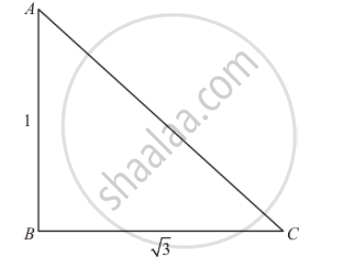Advertisement Remove all ads

# P the Ratio of the Length of a Rod and Its Shadow is 1 : √ 3 . the Angle of Elevation of the Sum is - Mathematics

MCQ

The ratio of the length of a rod and its shadow is 1 : sqrt3. The angle of elevation of the sum is

#### Options

• 30°

•  45°

• 60°

• 90°

Advertisement Remove all ads

#### Solution

Let θ be angle of elevation of sun.Given that: angle of elevation is 60°from tower of foot and distanceBC=100 meters.

Here, we have to find the height of tower.

So we use trigonometric ratios.

In a triangle ABC

⇒ tan C=( AB)/(BC)

⇒ tan 60°= (AB)/(BC)

⇒ sqrt 3=h/100

⇒=100sqrt3

Hence correct option is a.

Is there an error in this question or solution?
Advertisement Remove all ads

#### APPEARS IN

RD Sharma Class 10 Maths
Chapter 12 Trigonometry
Q 1 | Page 41
Advertisement Remove all ads

#### Video TutorialsVIEW ALL 

Advertisement Remove all ads
Share
Notifications

View all notifications

Forgot password?
Course